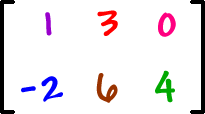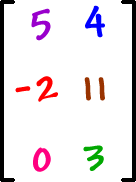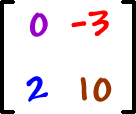So, this matrix...has 2 rows and 3 columns.
He's called a 2 x 3 matrix.

That's read "a 2 by 3 matrix" and that's NOT a multiplication sign!

What's the size of this matrix?3 rows and 2 columns...
This is a
3 x 2 matrix.

* A 2 x 3 matrix and a 3 x 2 matrix are definitely different sizes!

This guy is a 2 x 2 matrix:He's a square matrix.

We'll be able to do some special things with square matrices that we won't be able to do with other sizes.  (For example, we can only take determinants of square matrices.)